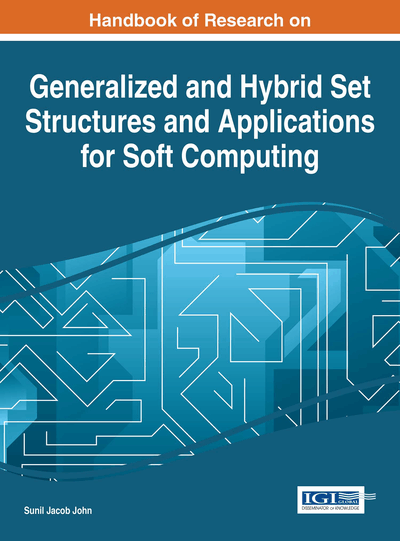# Rough Approximations on Hesitant Fuzzy Sets

D. Deepak (National Institute of Technology Calicut, India) and Sunil Jacob John (National Institute of Technology Calicut, India)
DOI: 10.4018/978-1-4666-9798-0.ch013

## Abstract

Introduction of hesitant fuzzy rough sets would facilitate the use of rough set based techniques to hesitant fuzzy environment. Hesitant fuzzy rough sets deal with the lower and upper approximations in a hesitant fuzzy domain. For this purpose concepts of hesitant fuzzy relations are discussed first to create a theoretical framework to study hesitant fuzzy rough sets. The concepts of equivalence relations are discussed. Hesitant fuzzy rough sets and the properties of the approximations are discussed. The dual nature of the lower and upper approximations is proved. This chapter introduces the model of a hesitant fuzzy rough set which approximates a hesitant fuzzy set using a hesitant fuzzy relation.
Chapter Preview
Top

## 1. Introduction

Characterization of uncertainty is a major research area in Soft Computing. Rough set based techniques to characterise uncertainty have been used efficiently in many areas including reasoning from imprecise data. Rough set theory, introduced by Pawlak (1982), consists of the approximation of the set by its lower and upper approximations. It uses the notion of an indiscernibility relation to partition the universal set to equivalence classes called granules. The lower approximation consists of all those granules which completely belong to the set whereas the upper approximation consists of elements with non empty intersection. The difference between the upper approximation and the lower approximation forms the boundary region thereby characterizing the uncertain elements. But the notion of an indiscernibility relation is very much restrictive when it comes to applications and further scope of the area. It is here that the importance of hybrid structures involving rough sets comes to play. Pawlak (2004) and Pawlak (1991) discuss concepts regarding rough sets and reasoning about data in great detail.

Zadeh (1965) introduced fuzzy sets which helped in characterising uncertainty by allotting membership values to elements in the set. Various other developments to these concepts have been experimented with great success. Atanassov (1986) introduced intuitionistic fuzzy sets which could additionally account for non membership values of the elements. Hai-long Sheng-gang (2009) discusses the intuitionistic fuzzy relations in great detail. Hesitant fuzzy sets introduced by Torra (2010), Torra and Narukawa (2009) assigns a set of possible membership values to every element in the set. The set of possible membership values arises because of difficulties in assigning a certain membership value to the element. The difficulties might arise due to many reasons. It may be the lack of knowledge or for example in decision making problems; situations may arise where the decision makers are not able to arrive at a mutually acceptable decision. Hesitant fuzzy sets opened up new areas of research by allowing scope for hesitancy in the membership values of a set.

Hybrid structures involving fuzzy sets and rough sets have also been studied in great detail by many researchers. Dubois and Prade (1990) studies rough fuzzy sets and fuzzy rough sets effectively. Nanda and Majumdar (1992) discuss various theoretical aspects regarding fuzzy rough sets. Wei et al. (2012) have analysed the relationships among rough approximations of some of the generalized rough set models for hybrid data. Variable precision fuzzy rough set model, studied by Mieszkowicz and Rolka (2008), is one of the suitable tools for analyzing information systems with crisp or fuzzy attributes. Hassanien (2007) introduced a hybrid scheme that combines the advantages of fuzzy sets and rough sets in conjunction with statistical feature extraction techniques the use of which has been demonstrated for breast cancer detection (by classifying the breast cancer images). Some shortcomings of traditional rough set attribute reduction, dealing with noise and real-valued attributes, have been addressed to a certain extend by Jensen and Shen (2004) by presenting a fuzzy-rough method for attribute reduction. Intuitionistic fuzzy rough relations and their properties have been studied in detail by Mukherjee and Das (2013). Degang and Suyun (2010) emphasised the use of local reduction with fuzzy rough sets for decision systems. Petrosino and Ferone (2009) have effectively used the properties and operations of rough fuzzy sets for the introduction of a new coding/decoding scheme for image compression.

## Key Terms in this Chapter

Fuzzy Set: An extension of a classical notion of a set whose elements have degrees of membership.

Hesitant fuzzy relation: Hesitant fuzzy subset of the Cartesian product of several crisp sets.

Equivalence Hesitant Fuzzy Relation: Hesitant fuzzy relation that is reflexive symmetric and transitive.

Hesitant Fuzzy Set: A set with elements having membership from the power set of [0,1].

Symmetric Kernel: Maximal symmetric hesitant fuzzy relation contained in any given hesitant fuzzy relation R .

Rough Set: An approximation of a set in terms of a pair of sets called the lower approximation and the upper approximation. The set difference between the lower and the upper approximations gives the boundary region which characterises the uncertainty.

Anti-reflexive Kernel: Maximal anti-reflexive hesitant fuzzy relation contained in any given hesitant fuzzy relation R .

Anti-reflexive Relation: A hesitant fuzzy relation R with the property R ( x,y ) = {0} ? x ? X .

Symmetric Hesitant Fuzzy Relation: A hesitant fuzzy relation R with the property R = R -1 .

Hesitant Fuzzy Rough Set: A hybrid set structure involving hesitant fuzzy sets and rough sets.

## Complete Chapter List

Search this Book:
Reset